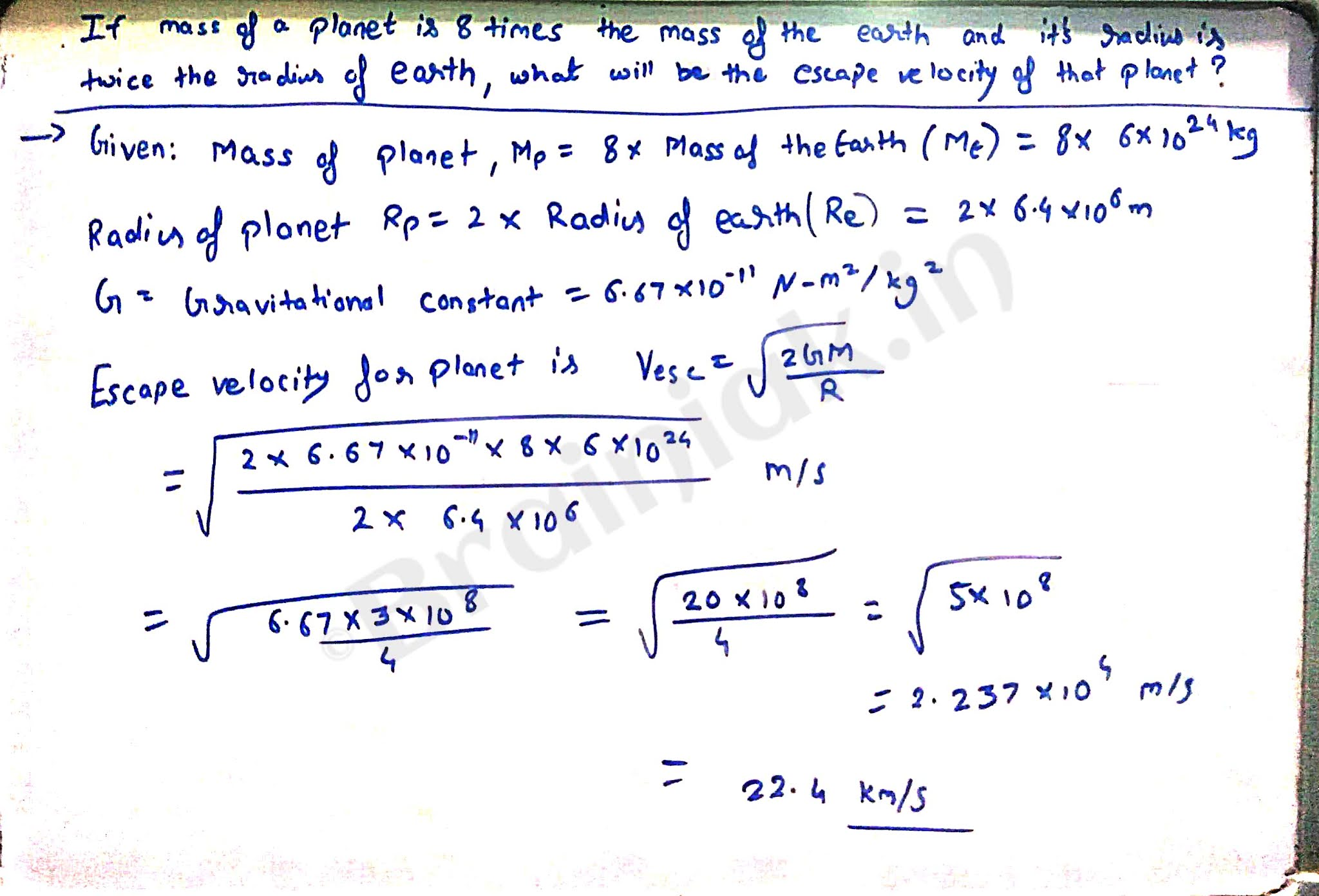If mass of a planet is eight times the mass of the earth and its radius is twice the radius of the earth, what will be the escape velocity for that planet?

If mass of a planet is eight times the mass of the earth and its radius is twice the radius of the earth, what will be the escape velocity for that planet?

verified